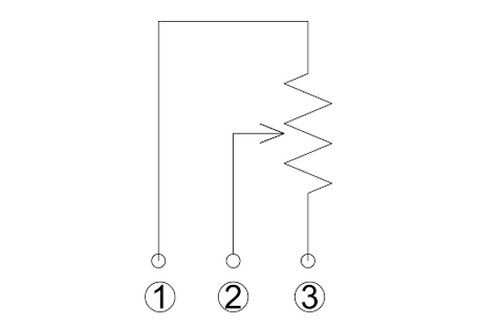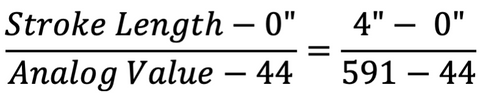# Potentiometer Feedback from a Linear Actuator With Video

## Potentiometers

Potentiometers, which are variable resistors, are used in linear actuators to provide positional feedback base on how their resistance changes. Linear actuators that use potentiometers for feedback, like our Feedback Rod Linear Actuator, will consist of the 3 additional wires like shown below, wire 1 for the input voltage, wire 2 is the variable resistor, and wire 3 is for ground. You can read the output of the potentiometers by measuring the voltage between wire 2, the output, and ground, which will provide an indication of the absolute position of the linear actuator. To utilize this feedback, you’ll need to make use of some type of microcontroller, like an Arduino, to read this positional value as the actuator moves.## Positional Feedback from a Potentiometer

As the variable resistor of the potentiometer changes as the linear actuator moves, the voltage difference between wire 2 and ground will change. Because of this, the feedback can easily be handled in the software of a controller, like an Arduino, as you can simply compare the output voltage of wire 2 to ground. Using an Arduino, this can simply be done by using an analog in pin of a microcontroller and using the analogRead() function to read the voltage from wire 2. An example of how to connect an Arduino to a linear actuator with potentiometer feedback is shown below.The analog in pins of the Arduino are analog-to-digital converters (ADC), which will convert the analog voltage on wire 2 to a 10-bit ADC value that will be between 0 to 1023. A 10-bit ADC value means the converter will translate the analog signal into 2^10 or 1024 distinct values ranging from 0 to 1023. Not all microcontrollers are 10-bit ADC, some are 8-bit or 16-bit, and the greater the number of bits the greater the resolution of the ADC. Once you have converted the analog signal into a digital value, to determine a position value in terms of stroke length, like in inches, you’ll need to find the exact analog range of your particular linear actuator as it may not range from 0 to 1023. This is because of the gearbox inside the actuator preventing the potentiometer fully rotating to its limit and means you’ll need to determine this range manually. For the coding example below, the 4” stroke length Feedback Rod Linear Actuator had an analog value of 44 at 0” and an analog value of 951 at 4”. Using these values, you can use ratios to determine the stroke length value like below:This can be simplified into Stroke Length = 0.00441*(Analog Value – 44), like in the coding example below. How often you read this analog value in the code of your microcontroller is another important consideration. In the code example below, the Arduino reads the potentiometer and updates the position value as long as the actuator is moving. But you could also make use of internal timers to update the position value over a set interval of time or you could simply place the potentiometer read function inside of the main loop of the code and continuously update the position value. Although the latter is not recommended if you plan to use your controller to perform multiple individual functions.

https://gist.github.com/OMikeGray/4dec9e075a8fe41efaea001fa1e98d70

## Dealing with Electrical Noise

One drawback of feedback from the potentiometer is that it can be affected by electrical noise and may make your positional value unstable. One way to overcome this is to use a digital filter to remove the electrical noise and achieve stable results. There are few different types of filters that can be used from exponential filters to high-pass and band-pass filters each with their own benefits, but for many applications with linear actuators, simply using a running average of the positional value will work. A running average is simply the average of the last X amount of measurements to smooth out the input signal. The exact number of measurements you want to average will depend on your application and you may need to play around with this number to determine what works best. A few things to be aware of, if you have too few measurements in your average, your signal will still be noisy, but if you have too many measurements, your results will lag too far behind the actual position of the actuator to be useable. It is finding a balance between too few and too many measurements that will make your filter effective. Below is a plot showing the effect of a running average filter versus the actual input signal.The code used to implement the running average is shown below, it uses an average of 3 measurements to smooth the input signal. Three measurements were chosen because there wasn’t a lot of noise in actual input signal, so only a few measurements were needed to smooth out the value. If there was more noise in the input signal, a larger number of measurements would be needed. In situations where there is a lot of inductive components (i.e. motors) electrical noise will be a much larger problem.

https://gist.github.com/OMikeGray/b13f156c080a100a89e5bbd541d0565e

## Utilizing Feedback for Automation